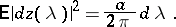# White noise

A generalized stationary stochastic processwith constant spectral density. The generalized correlation function of white noise has the form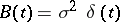, where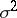is a positive constant and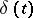is the delta-function. The white noise process is extensively applied in describing random disturbances with a very small correlation period (e.g. "thermal noisethermal noise" — pulsations of the current intensity in a conductor, generated by the thermal motion of the electrons). In the spectral decomposition of white noise,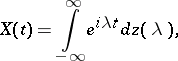the "elementary vibrations"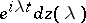have, on the average, the same intensity at all frequencies; more accurately, their average squared amplitude is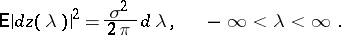This spectral decomposition means that, for each square-integrable function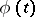,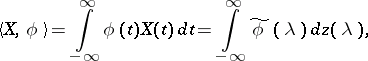where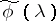is the Fourier transform of; a more explicit dependence of the generalized processon the functionmay be described by a corresponding stochastic measure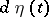of the same type as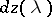(is the Fourier transform of the stochastic measure), viz.Gaussian white noise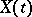, which is the generalized derivative of Brownian motion(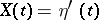), is the basis for constructing stochastic diffusion processes(cf. Diffusion process), "controllable" by a stochastic differential equation: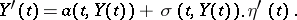These equations are often written in the form of differentials:Another important model involving the use of white noise is the stochastic process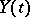which describes the behaviour of a stable vibrating system acted upon by stationary random perturbations, when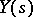,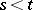, do not depend on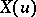,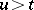. A very simple example of this is the system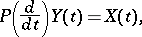where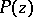is a polynomial with roots in the left half-plane; after the damping of the "transient processes" , the processis given by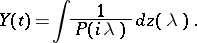In practical applications, in the description of the so-called shot effect process, white noise of the form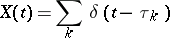plays an important role (varies betweenandand the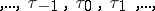form a Poisson process); more accurately,is the generalized derivative of a Poisson process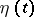. The shot effect process itself has the form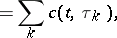where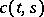is some weight function satisfying the condition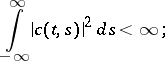in addition, the average value of the generalized processis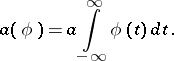Here,is the parameter of the Poisson law (see above), and the stochastic measurein the spectral representationof this process is such that